### Marginal Likelihood

Based on the model that is specified in the section Notation for the Item Response Theory Model, the marginal likelihood is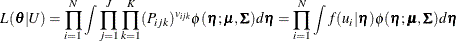where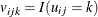,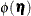is the multivariate normal density function for the latent factor
, andis a set of all the model parameters. The corresponding log likelihood is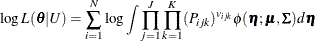Integrations in the preceding equation cannot be solved analytically and need to be approximated by using numerical integration,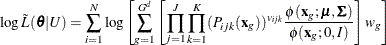where d is the number of factors, G is the number of quadrature points per dimension, and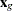and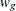are the quadrature points and weights, respectively.